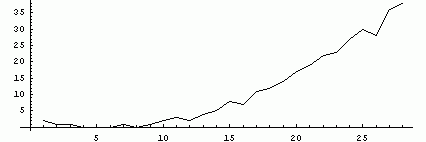## Power Sums

Ed Pegg Jr., November 13, 2006

In 1772, Euler made a conjecture: If a sum of n positive kth powers equals one kth power, then nk.

In 1966, Lander, Parkin and Selfridge proved Euler wrong with 144= 1335+1105+845+275. Let this solution be considered as (5.1.4). All parts are 5th powers, and 1 of them is equal to 4 others, or (5.1.4). Lander, Parkin and Selfridge further conjectured that for other powers sums (k,m,n), that m+nk. So far, no counterexamples have been found. Only seven forms are known with equality: 4.1.3, 4.2.2, 5.1.4, 5.2.3, 6.3.3, 8.3.5, and 8.4.4. The last of these was discovered by Nuutti Kuosa on Nov 9, 2006.

1584+594 = 1344+1334 (4.2.2) infinite solutions
4224814=4145604+2175194+958004
(4.1.3) infinite solutions
1445 = 1335+1105+845+275
(5.1.4) 2 known solutions
141325+2205 = 140685+62375+50275
(5.2.3) 1 known solution
236+156+106 = 226+196+36
(6.3.3) 10 known solutions
9668+5398+818 = 9548+7258+4818+3108+1588
(8.3.5) 1 known solution
31138+20128+19538+8618=28238+27678+25578+11288
(8.4.4) 1 known solution
Figure 1. Known solutions for (k,m,n) with m+n = k.

Since March 1999, such results have been collected by EulerNet, headed by programmer Jean-Charles Meyrignac. Over 200 EulerNet contributors have put in almost 200 years of computer time looking for solutions, and improving search methods. Many of the solutions above are due to the EulerNet group. There are no known equalities for 6.1.5, 6.2.4, 7.1.6, 7.2.5, 7.3.4, 8.1.7, and 8.2.6, or for any higher powers. A plot of the excess for the best known solutions is below:Figure 2. Excess of best known solutions. Only 4,5,6, and 8 have solutions with 0 excess.

Another power sum problem is the Fermat-Catalan conjecture, which claims there are a finite number of solutions to xp+yq = zr, when 1/p + 1/q + 1/r < 1. There are ten known solutions, excluding trivial variations of the first example.

17+23 = 32
25+72 = 34
73+132 = 29
27+173 = 712
35+114 = 1222
14143+22134592 = 657
338+15490342 = 156133
92623+153122832 = 1137
438+962223 = 300429072
177+762713 = 210639282

Figure 3. Known solutions for the Fermat-Catalan conjecture.

A third power problem is the abc-conjecture. Consider a number that is a power, or which contains many powers. rad(n) is the product of all distinct prime divisors of n. For example, if n = 32×56×73, then rad(n) is 3×5×7, or 105. For sums a+b=c, there are only seven known examples where log(c)/log(rad(abc)) > 1.54.

2 + 310×109 = 235
112 + 32×56×73 = 221×23
19×1307 + 7×292×318 = 28×322×54
283 + 511×132 = 28×38×173
1 + 2×37 = 54×7
73 + 310 = 211×29
72×412×3113 + 1116×132×79 = 2×33×523×953
Figure 4. Known solutions for log(c)/log(rad(abc)) > 1.54 in the abc-conjecture.

An alternate form of the problem is to consider log(abc)/log(rad(abc)). Only ten examples are known where log(abc)/log(rad(abc))>4.2.

13×196 + 230×5 = 313×112×31
25×112×19+ 515×372×47  = 37×711×743
219×13×103 + 711 = 311×53×112
198×434×1492 + 215×523×101 = 313×13×292×376×911
235×72×172×19 + 327×1072  = 515×372×2311
318×23×2269 + 173×29×318 = 210×52×715
174×793×211 + 229×23×292 = 519
514×19 + 25×3×713 = 117×372×353
27×54×722 + 194×37×474×536 = 314×11×139×191×7829
321 + 72×116×199 = 2×138×17
Figure 5. Known solutions for log(abc)/log(rad(abc)) > 4.2 in the abc-conjecture.

All of these problems are considered extremely difficult, so many congratulations to Nuutti Kuosa for extending one of these lists.

References:

Richard Guy, Unsolved Problems in Number Theory, Springer, 2004.

Jean-Charles Meyrignac, EulerNet, "Computing Minimal Equal Sums Of Like Powers" http://euler.free.fr/.